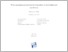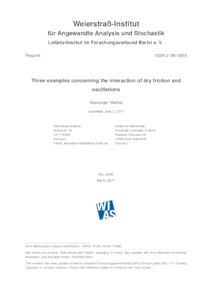Repository: Freie Universität Berlin, Math Department

# Three examples concerning the interaction of dry friction and oscillations

Mielke, A. (2017) Three examples concerning the interaction of dry friction and oscillations. In: Trends on Application of Mathematics to Mechanics. Springer Proceedings of the INdAM-ISIMM Workshop, Rome, Italy, September 2016 (27). Springer INdAM series, pp. 159-177.Preview

3MB

## Abstract

We discuss recent work concerning the interaction of dry friction, which is a rate independent effect, and temporal oscillations. First, we consider the temporal averaging of highly oscillatory friction coefficients. Here the effective dry friction is obtained as an infimal convolution. Second, we show that simple models with state-dependent friction may induce a Hopf bifurcation, where constant shear rates give rise to periodic behavior where sticking phases alternate with sliding motion. The essential feature here is the dependence of the friction coefficient on the internal state, which has an internal relaxation time. Finally, we present a simple model for rocking toy animal where walking is made possible by a periodic motion of the body that unloads the legs to be moved.

Item Type: Book Section Preprint 06/2017: WIAS Preprint No. 2405 Mathematical and Computer Sciences > Mathematics > Applied Mathematics Department of Mathematics and Computer Science > Institute of Mathematics 2175 Silvia Hoemke 08 Jan 2018 10:34 02 Mar 2021 15:11

Repository Staff Only: item control page